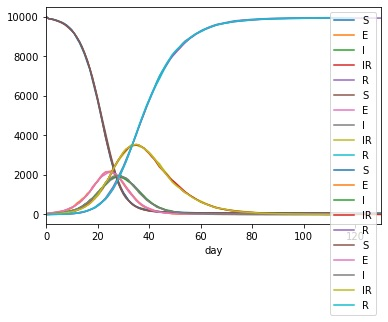# Extending the Model in Python¶

## Adding a disease stage¶

Continuing in ipython/jupyter from the last session, we will now extend the disease to include an additional, less-infectious, semi-recovering stage, which will come after I, and be called IR. We do this by inserting a new stage, named “IR”, at index 2, with `beta` value 0.2, and `progress` value 0.1

```>>> lurgy.insert(2, name="IR", beta=0.2, progress=0.1)
>>> print(lurgy)

* Disease: lurgy
* stage: ['E', 'I', 'IR', 'R']
* mapping: ['E', 'I', 'IR', 'R']
* beta: [0, 0.8, 0.2, 0]
* progress: [0.25, 0.25, 0.1, 0]
* too_ill_to_move: [0, 0, 0.0, 0]
* start_symptom: 2
```

Note

MetaWards is a Python program, so the index is counted from 0. Index 0 is E, index 1 is I and (before this call), index 2 was R. Inserting at index 2 will insert IR between I and R

We can now run the model using `metawards.run()`. This time we will set `silent` to `TRUE` so that it doesn’t print so much output to the screen.

```>>> results = mw.run(model=home, disease=lurgy,
additional=100, silent=True)

━━━━━━━━━━━━━━━━━━━━━━━━━━━━━━━━━━━━━ INFO ━━━━━━━━━━━━━━━━━━━━━━━━━━━━━━━━━━━━━
Writing output to directory ./output_s839le7f

━━━━━━━━━━━━━━━━━━━━━━━━━━━━━━━━━━━━━━━━━━━━━━━━━━━━━━━━━━━━━━━━━━━━━━━━━━━━━━━━
```

Note

All of the output is written to the (randomly) named output directory indicated, e.g. for me to output_s839le7f. The full log of the run is recorded in the file called `console.log.bz2` which is in this directory.

We can now process and plot the results similarly to before, e.g.

```>>> df = pd.read_csv(results)
>>> df.plot.line(x="day", y=["S","E","I","IR","R"])
```

## Repeating a run¶

MetaWards model runs are stochastic, meaning that they use random numbers. While each individual run is reproducible (given the same random number seed and number of processor threads), it is best to run multiple runs so that you can look at averages.

You can perform multiple runs using the `repeats` argument, e.g. to perform four runs, you should type;

```>>> results = mw.run(model=home, disease=lurgy,
additional=100, silent=True, repeats=4)
```

If you look at the results, you will that there is a repeat column, which indexes each run with a repeat number, e.g.

```>>> df = pd.read_csv(results)
>>> print(df)

fingerprint  repeat  day        date      S   E   I  IR     R  IW
0        REPEAT       1    0  2020-07-22  10000   0   0   0     0   0
1        REPEAT       1    1  2020-07-23   9900  88  12   0     0   1
2        REPEAT       1    2  2020-07-24   9890  74  30   6     0   1
3        REPEAT       1    3  2020-07-25   9864  83  40  13     0   1
4        REPEAT       1    4  2020-07-26   9835  95  48  20     2   1
..          ...     ...  ...         ...    ...  ..  ..  ..   ...  ..
553      REPEAT       4  148  2020-12-17     63   0   0   1  9936   0
554      REPEAT       4  149  2020-12-18     63   0   0   1  9936   0
555      REPEAT       4  150  2020-12-19     63   0   0   1  9936   0
556      REPEAT       4  151  2020-12-20     63   0   0   1  9936   0
557      REPEAT       4  152  2020-12-21     63   0   0   0  9937   0
```

We can group by repeat and plot using;

```>>> import matplotlib.pyplot as plt
>>> df.groupby("repeat").plot.line(
x="day", y=["S","E","I","IR","R"], ax=plt.gca())
```

You should get a result that looks something like this;Note

With a bit more pandas you can make this plot a lot prettier ;-)

From this you can see the build-up of individuals in the yellow long recovery (IR) stage.

## Adding more wards¶

Next, we will extend the model by adding more wards. We will model home, work and school, so let’s now add the work and school wards.

```>>> work = mw.Ward("work")
>>> school = mw.Ward("school")
```

We will now add some workers who will make daily, predictable movements from home to work or school.

```>>> home.add_workers(7500, destination=work)
>>> home.add_workers(5000, destination=school)
```

Note

The term worker is very broad in MetaWards. It means any individual that make regular, predictable movements each day. In this case, it refers to workers, teachers and students.

Next we need to combine these individual `Ward` objects into a single `Wards` that represents the entire network.

```>>> network = mw.Wards()
>>> network.add(home)
>>> network.add(work)
>>> network.add(school)
```

## Running the model¶

We can now run the model. In this case, we want to seed the infection in the home ward, so we need to pass this name into the `additional` parameter.

```>>> results = mw.run(disease=lurgy, model=network,
additional="1, 100, home")
```

Note

The format is day number (in this case seed on day 1), then number to seed (seeding 100 infections), then ward name or number (in this case, home)

You will see a lot of output. MetaWards does print a table to confirm the seeding, e.g.

```┏━━━━━┳━━━━━━━━━━━━━┳━━━━━━━━━━━━━━━━━━━━━━━━━━━━━━━━━━━━━━━━━━━━━━┳━━━━━━━━━━━┓
┃ Day ┃ Demographic ┃                     Ward                     ┃  Number   ┃
┃     ┃             ┃                                              ┃  seeded   ┃
┡━━━━━╇━━━━━━━━━━━━━╇━━━━━━━━━━━━━━━━━━━━━━━━━━━━━━━━━━━━━━━━━━━━━━╇━━━━━━━━━━━┩
│  1  │    None     │ 1 : WardInfo(name='home', alternate_names=,  │    100    │
│     │             │   code='', alternate_codes=, authority='',   │           │
│     │             │        authority_code='', region='',         │           │
│     │             │               region_code='')                │           │
└─────┴─────────────┴──────────────────────────────────────────────┴───────────┘
```

The results can be processed and visualised as before, e.g.

```>>> df = pd.read_csv(results)
>>> df.plot.line(x="day", y=["S","E","I","IR","R"])
```

## Complete code¶

The complete Python code for this part of the getting started guide is re-copied below (this continues from the code in the last part);

```# extend the disease model
lurgy.insert(2, name="IR", beta=0.2, progress=0.1)

# run the model
results = mw.run(model=home, disease=lurgy,
additional=100, silent=True)

# load and graph the results
df = pd.read_csv(results)
df.plot.line(x="day", y=["S","E","I","IR","R"])

# run multiple repeats
results = mw.run(model=home, disease=lurgy,
additional=100, silent=True, repeats=4)

# load and graph the results
df = pd.read_csv(results)
import matplotlib.pyplot as plt
df.groupby("repeat").plot.line(
x="day", y=["S","E","I","IR","R"], ax=plt.gca())

# add more wards
work = mw.Ward("work")
school = mw.Ward("school")
home.add_workers(7500, destination=work)
home.add_workers(5000, destination=school)

# build the network
network = mw.Wards()
network.add(home)
network.add(work)
network.add(school)

# run the model
results = mw.run(disease=lurgy, model=network,
additional="1, 100, home")

# load the graph the results
df = pd.read_csv(results)
df.plot.line(x="day", y=["S","E","I","IR","R"])
```